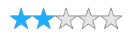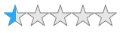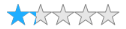# Precision in WPF Rating (SfRating)

7 May 20212 minutes to read

The precision mode defines the accuracy level of the SfRating control. It has Standard, Half, and Exact options. By default, the precision mode of the SfRating control is set to `Standard`.

### Standard

When the precision mode of the SfRating control is set to `Standard`, the rating item will be filled completely based on the rating value.

``<rating:SfRating ItemsCount="5" Precision="Standard"></rating:SfRating>``
``````SfRating rating;
public MainWindow()
{
InitializeComponent();
rating = new SfRating();
rating.ItemsCount = 5;
rating.Precision = Precision.Standard;
Content = rating;
}``````### Half

When the precision mode of the SfRating control is set to `Half`, the rating item will be filled partially based on the rating value.

``<rating:SfRating ItemsCount="5" Precision="Half"></rating:SfRating>``
``````SfRating rating;
public MainWindow()
{
InitializeComponent();
rating = new SfRating();
ItemsCount = 5;
rating.Precision = Precision.Half;
Content = rating;
}``````### Exact

If the precision mode of SfRating is set to `Exact`, the rating item will be filled exactly based on the rating value.

``<rating:SfRating ItemsCount="5" Precision="Exact"></rating:SfRating>``
``````SfRating rating;
public MainWindow()
{
InitializeComponent();
rating = new SfRating();
rating.ItemsCount = 5;
rating.Precision = Precision.Exact;
Content = rating;
}``````